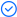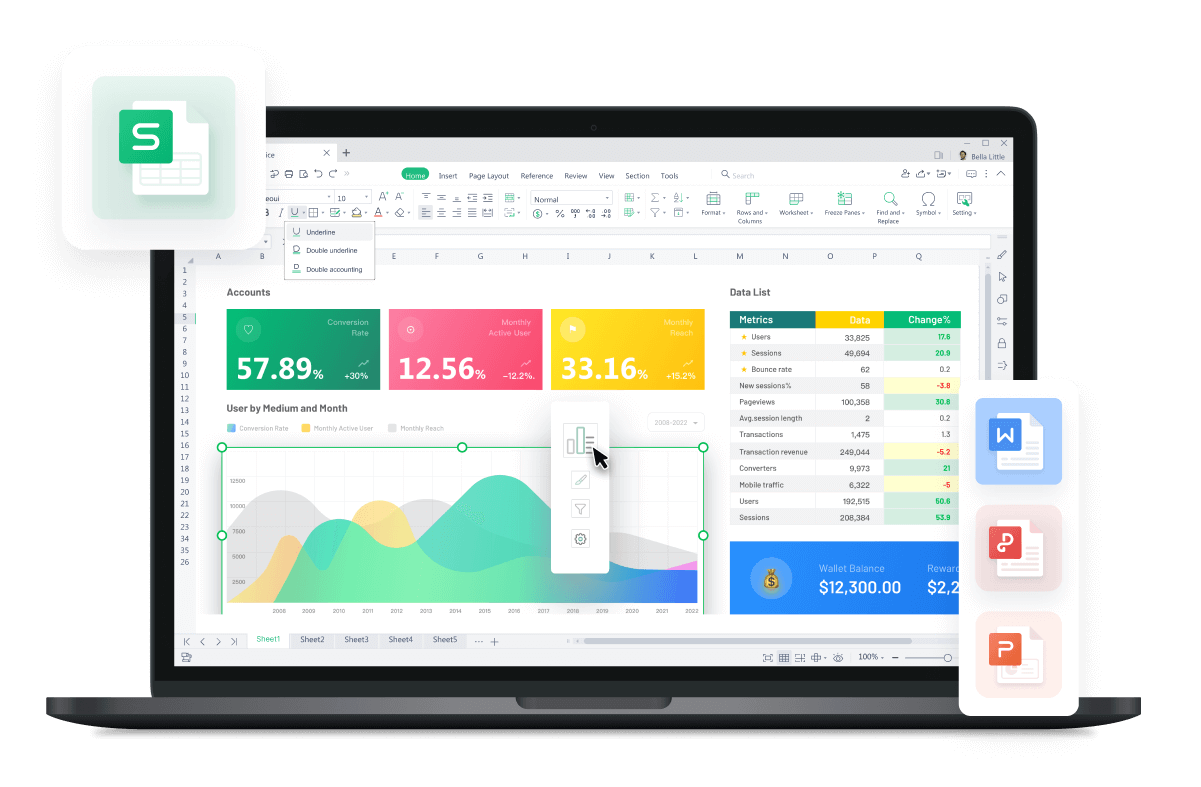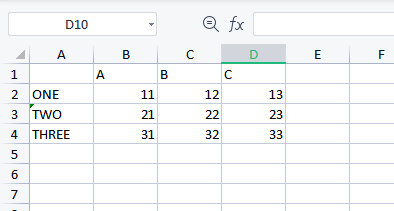WPS Office

Free All-in-One Office Suite with PDF EditorRead, edit, and convert PDFs with the powerful PDF toolkit.Microsoft-like interface, easy to use.

Windows • MacOS • Linux • iOS • Android# How Python read Excel files using Pandas

July 22, 2022
4.0K Views

Python read Excel files with the.xlsx and.xls extension. Use the read_excel()  function of the pandas library to read an excel file as a DataFrame.

You have a choice of reading the initial sheet, certain sheets, many pages, or all sheets. In this article, we will be learning about how Python read Excel file.

Make a spreadsheet with sheets 1 and 2 in it.

sheet: 01:sheet: 02:Internally, Pandas. .read_excel a.) makes use of the xlrd library.

Python read Excel file (.xlsx,.xls) using the xlrd package.

How Python read Excel file using xlrd and xlwt:

An error message resembling the following may appear if you attempt to use pandas.read excel s() in a setting where xlrd is not present:

ImportError: Install xlrd >= 0.9.0 for Excel support

Pip may be used to install xlrd.

\$ pip install xlrd

View excel:

Put the Excel file's path or URL in the first parameter. Pandas only use the first sheet when there are many sheets. It is called DataFrame.

import pandas as pd

print(df)

The excel sheet's content is produced using the code above:

Unnamed: 0   A   B   C
0        one  11  12  13
1        two  21  22  23
2      three  31  32  33

Take a sheet:

With the option sheet name, you may indicate which sheet should be read. Indicate by number (starting at 0).

print(df_sheet_index)

 #        AA  BB  CC# ONE    11  12  13# TWO    21  22  23# THREE  31  32  33

Specify by sheet name: df_sheet_name = pd.read_excel('sample.xlsx', sheet_name='sheet2')

print(df_sheet_name)

 #        AA  BB  CC# ONE    11  12  13# TWO    21  22  23# THREE  31  32  33

Python Read Several sheets: A list can also be specified in the argument sheet name. Even a number with a 0 as the first digit of the sheet name is acceptable. The key key and the data pandas are the given number or sheet name. The ordered dictionary OrderedDict with the value value is read from the DataFrame. df_sheet_multi = pd.read_excel('sample.xlsx', sheet_name=[0, 'sheet2'])

print(df_sheet_multi)

 print(df_sheet_multi)#         A   B   C# one    11  12  13# two    21  22  23# three  31  32  33print(type(df_sheet_multi))#print(df_sheet_multi['sheet2'])#        AA  BB  CC# ONE    11  12  13# TWO    21  22  23# THREE  31  32  33print(type(df_sheet_multi['sheet2']))#

Python Read All Sheet: All sheets are read if the sheet name parameter is null. df_sheet_all = pd.read_excel('sample.xlsx', sheet_name=None)
print(df_sheet_all)

In this case, the sheet name becomes the key.

 print(df_sheet_all['sheet1'])#         A   B   C# one    11  12  13# two    21  22  23# three  31  32  33print(type(df_sheet_all['sheet1']))#print(df_sheet_all['sheet2'])#        AA  BB  CC# ONE    11  12  13# TWO    21  22  23# THREE  31  32  33print(type(df_sheet_all['sheet2']))#